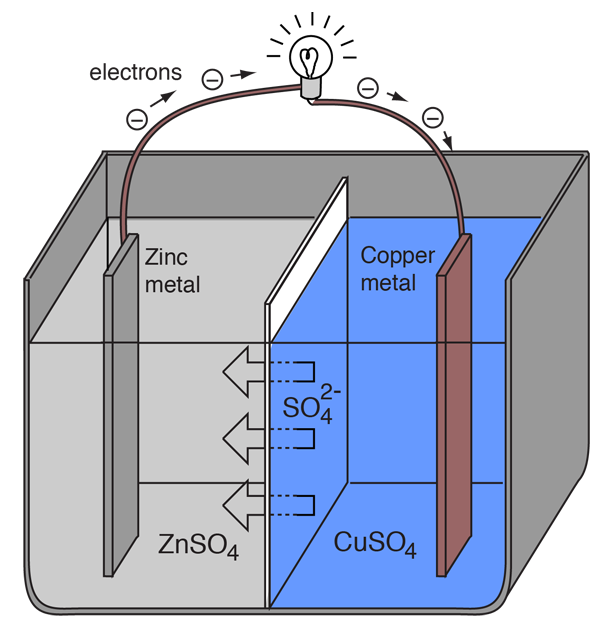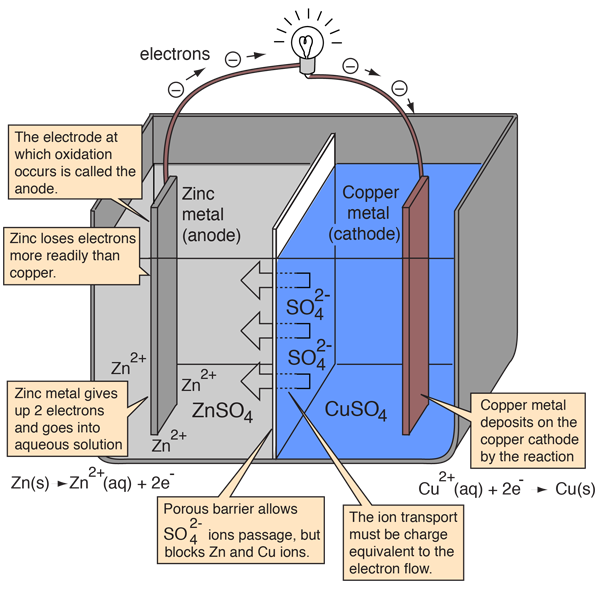Electrochemical Cells

An extremely important class of oxidation and reduction reactions are used to provide useful electrical energy in batteries. A simple electrochemical cell can be made from copper and zinc metals with solutions of their sulfates. In the process of the reaction, electrons can be transferred from the zinc to the copper through an electrically conducting path as a useful electric current.

An electrochemical cell can be created by placing metallic electrodes into an electrolyte where a chemical reaction either uses or generates an electric current. Electrochemical cells which generate an electric current are called voltaic cells or galvanic cells, and common batteries consist of one or more such cells. In other electrochemical cells an externally supplied electric current is used to drive a chemical reaction which would not occur spontaneously. Such cells are called electrolytic cells.

Index

Oxidation/
Reduction concepts

Electrochemistry concepts

Reference
Hill & Kolb
Ch 8

Ebbing
Ch 19

 HyperPhysics***** Electricity and Magnetism ***** Chemistry R Nave
Go Back

Voltaic Cells

An electrochemical cell which causes external electric current flow can be created using any two different metals since metals differ in their tendency to lose electrons. Zinc more readily loses electrons than copper, so placing zinc and copper metal in solutions of their salts can cause electrons to flow through an external wire which leads from the zinc to the copper.As a zinc atom provides the electrons, it becomes a positive ion and goes into aqueous solution, decreasing the mass of the zinc electrode. On the copper side, the two electrons received allow it to convert a copper ion from solution into an uncharged copper atom which deposits on the copper electrode, increasing its mass. The two reactions are typically written

Zn(s) -> Zn2+(aq) + 2e-

Cu2+(aq) + 2e- -> Cu(s)

The letters in parentheses are just reminders that the zinc goes from a solid (s) into a water solution (aq) and vice versa for the copper. It is typical in the language of electrochemistry to refer to these two processes as "half-reactions" which occur at the two electrodes.

Zn(s) -> Zn2+(aq) + 2e-

 The zinc "half-reaction" is classified as oxidation since it loses electrons. The terminal at which oxidation occurs is called the "anode". For a battery, this is the negative terminal.
 The copper "half-reaction" is classified as reduction since it gains electrons. The terminal at which reduction occurs is called the "cathode". For a battery, this is the positive terminal.

Cu2+(aq) + 2e- -> Cu(s)

In order for the voltaic cell to continue to produce an external electric current, there must be a movement of the sulfate ions in solution from the right to the left to balance the electron flow in the external circuit. The metal ions themselves must be prevented from moving between the electrodes, so some kind of porous membrane or other mechanism must provide for the selective movement of the negative ions in the electrolyte from the right to the left.

Energy is required to force the electrons to move from the zinc to the copper electrode, and the amount of energy per unit charge available from the voltaic cell is called the electromotive force (emf) of the cell. Energy per unit charge is expressed in volts (1 volt = 1 joule/coulomb).

Clearly, to get energy from the cell, you must get more energy released from the oxidation of the zinc than it takes to reduce the copper. The cell can yield a finite amount of energy from this process, the process being limited by the amount of material available either in the electrolyte or in the metal electrodes. For example, if there were one mole of the sulfate ions SO42- on the copper side, then the process is limited to transferring two moles of electrons through the external circuit. The amount of electric charge contained in a mole of electrons is called the Faraday constant, and is equal to Avogadro's number times the electron charge:

Faraday constant = F = NAe = 6.022 x 1023 x 1.602 x 10-19 = 96,485 Coulombs/mole

The energy yield from a voltaic cell is given by the cell voltage times the number of moles of electrons transferred times the Faraday constant.

Electrical energy output = nFEcell

The cell emf Ecell may be predicted from the standard electrode potentials for the two metals. For the zinc/copper cell under the standard conditions, the calculated cell potential is 1.1 volts.

Index

Oxidation/
Reduction concepts

Electrochemistry concepts

Reference
Hill & Kolb
Ch 8

Ebbing
Ch 19

 HyperPhysics***** Electricity and Magnetism ***** Chemistry R Nave
Go Back

Details of the Daniell Cell

English chemist John Frederick Daniell developed a voltaic cell in 1836 which used zinc and copper and solutions of their ions.Voltaic cell discussion
Index

Oxidation/
Reduction concepts

Electrochemistry concepts

Reference
Hill & Kolb
Ch 8

Ebbing
Ch 19

 HyperPhysics***** Electricity and Magnetism ***** Chemistry R Nave
Go Back